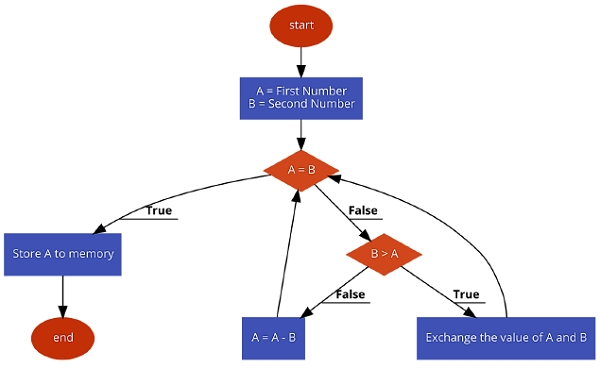# 8085 Program to find the HCF of two given bytes

In this program we will see how to find the HCF or GCD of two numbers using 8085.

### Problem Statement

Write 8085 Assembly language program to find the HCF of two numbers stored at memory location 8000H and 8001H.

### Discussion

This problem is solved by the Euclidean algorithm to find HCF. This algorithm is very simple.The algorithm steps are as follows −

• If first number and second number are same, then

• go to step 3.

Else if first number < second number, then

• exchange no1 andno2.
• first-number <-first-number – second-number; go to step 1

• Display result as first-number

The values are stored at location 8000H and 8001H, and the result will be stored at location8050H.

First Input

......
80002D
800169
......
80500F
......

Second Input

......
800025
800135
......
805001
......

### Flow Diagram### Program

F00021, 00, 80
LXI H,8000HPoint to the first number
F0037E
MOV A, MLoad the first number into Acc
F00423
INX H   Point to next location
F00546
MOV B, MLoad the second number
F006B8LOOPCMP B   Compare B with A
F007CA, 17, F0
JZ STORE    When A and Bare same, store the result
JC EXG   If B > A, then exchange B and A
F00D90
SUB B   if B < A,subtract B from A
F00EC3, 06, F0
F01247
MOV B, AMove A to B
F01379
MOV A, CMove C to A
F014C3, 06, F0
F01732, 50, 80STORESTA 8050H   Store the value into memory
F01A76
HLTTerminate the program

First Output